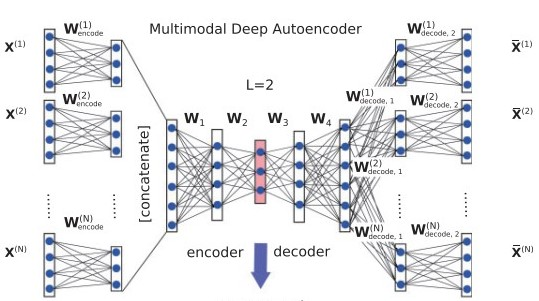# An autoencoder with multiple inputs

Hi everybody,
I’m new to pytorch and trying to implement a multimodal deep autoencoder(means: autoencoder with multiple inputs)
At the first all inputs encode with same encoder architecture, after that, all outputs concatenates together and the output goes into the another encoding and deoding layers:At the end, last decoder layer must reconstruct the inputs as multiple outputs.

Now I have between one and 9 inputs depending on the user’s choice and each input is a 1215x1519 matrix.

I’m rally stuck in first and last layers of this autoencoder.

Can anyone help me in this case?
Thanks.

@ptrblck

You could implement the posted model architecture using `nn.ModuleList`s as seen here:

``````class MyModel(nn.Module):
def __init__(self):
super().__init__()
self.encoders = nn.ModuleList()
for _ in range(9):
self.encoders.append(nn.Linear(4, 3))

self.encoder = nn.Sequential(
nn.Linear(9*3, 4),
nn.ReLU(),
nn.Linear(4, 3)
)
self.decoder = nn.Sequential(
nn.Linear(3, 4),
nn.ReLU(),
nn.Linear(4, 9*3)
)
self.decoders = nn.ModuleList()
for _ in range(9):
self.decoders.append(nn.Linear(3, 4))

def forward(self, inputs):
out = []
for idx, enc in enumerate(self.encoders):
out.append(enc(inputs[idx]))
out = torch.cat(out, dim=1)
z = self.encoder(out)
out = self.decoder(z)
out = torch.split(out, 3, dim=1)
outs = []
for idx, dec in enumerate(self.decoders):
outs.append(dec(out[idx]))
return outs

model = MyModel()
inputs = [torch.randn(1, 4) for _ in range(9)]
outs = model(inputs)
``````

I don’t know which activation functions should be used etc. so you could use this code snippet as a base implementation and adapt it to your use case.

Do you mean that you want to use the same model in case there are 5 inputs( each input being 1215x1519) or 1 input or 9 inputs?

Thank you for your help, it was very helpfulI have another problem:
Each input is a computed embedding of a graph and as I said before, each input is 1215x1519 matrix.
On the other hand, since we do not have any labels for our data, the original 9x1215x1519 (9 is number of inputs) data considered as label and then considered a noisy version of original data with same shape for model input, in this way we’re trying to reconstruct input according to the labels.

In another implementation of this case with tensorflow and keras, developer fit the model with keras fit() function:

##### For fitting the model
``````history = model.fit(X_train_noisy, X_train, epochs=epochs, batch_size=batch_size, shuffle=True,
validation_data=(X_test_noisy, X_test),
callbacks=[EarlyStopping(monitor='val_loss', min_delta=0.0001, patience=5)])
``````

Both X_train_noisy and X_train are 9x1215x1519.

Now I’m really confused about how to do this with pytorch!
Thank you again

I don’t know what exactly the Keras model is doing, as the `fit` method doesn’t show any information about the loss function etc.
You could thus check its internal implementation and use the same approach in PyTorch. E.g. if it’s some form of mse loss, use `nn.MSELoss` in PyTorch to calculate the loss.